## Chapter 2. Basic Concepts in Volumizer

This chapter describes the central concepts in Volumizer in the following sections:

The next chapter describes the API calls associated with these concepts.

## Volumetric vs. Surface-Based Models

In computer graphics, a three-dimensional object is any object that exists in three-dimensional space. Strictly speaking, however, the triangles and other surface elements used to represent such objects are two-dimensional primitives.

Figure 2-1. Geometric Primitives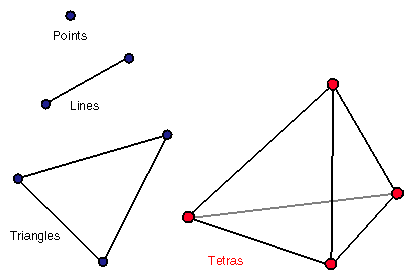Two-dimensional primitives suffice in many cases because most objects around us are adequately represented by their surface. Objects with interesting interiors, however, are abundant in everyday life. Clouds, smoke, and anatomy are all examples of volumetrically interesting objects.

Abstract volumetric objects, such as medical (CT, MRI, PET), geophysical, and computational data sets also contain interesting interior information that cannot easily be represented by surfaces, as shown in Figure 2-2.

Figure 2-2. Abstract Data Set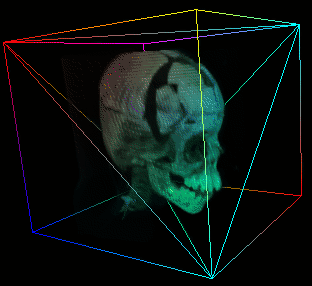Despite their abundance and importance, volumetric objects are either not handled at all or their treatment is substantially different from that of surface-based models. Handling heterogeneous scenes that contain volumes and surfaces, as a result, is very challenging and often done as a special case.

OpenGL Volumizer extends the concepts of surface-based models to include volumetric shapes. As a result, Volumizer arrives at a single, unified framework capable of handling both types of models equally well.

### Volumetric Properties

A volumetric model is not hollow; it has some property—for example, color or opacity—that varies throughout the interior of the object. Consider a color cube represented using two- and three-dimensional primitives:

• Traditionally, a colored surface-only cube is rendered by drawing six polygonal faces and interpolating the colors across each face.

• A colored volumetric cube interpolates the colors across the entire space occupied by the cube.

These two cubes look identical from the outside. However, the differences become apparent as soon as we try to fly through such object, or make the surface semi-transparent.

Figure 2-3. Colored Cubes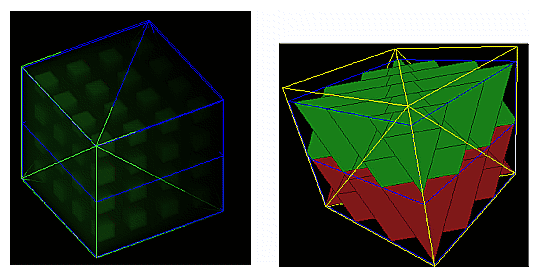## Decoupling Geometry and Appearance

In traditional three-dimensional graphics, graphical objects, called models or shapes in this document, are commonly described in terms of geometry and appearance. For example, a rectangular shape may be described by a quad mesh (geometry) with a two-dimensional texture (appearance) mapped onto it. In many cases there is a close relationship between appearance and geometry. For example, two-dimensional texture maps, which are rectangular arrays of values (pixels), are often mapped onto a single rectangular polygon of equal size.

Having an appearance match the size of a geometry, however, is a special, not a general case. For example, it is possible to move a circular geometry around inside a (larger) texture image like a magnifying glass, or to texture map an image onto a (smaller) sphere or a bicubic patch capable of squashing and twisting. In these examples, the shape's geometry and appearance are clearly decoupled.

It is the combination of geometry (for example, a sphere) and appearance (for example, voxels representing your data) that compose a volumetric shape. In the remainder of the book, the term “volume” describes the pairing of geometry and appearance.Note: Although a geometry can be drawn without a texture, a texture cannot be drawn directly; it can only be used to modify the way a geometry is rendered. This is in stark contrast to conventional volume rendering techniques, which consider a voxel to be a drawable entity by itself.

In the simplest case, a volume's dimensions match that of its data cube. In general, however, the dimensions of a volume and its appearance differ. Conventional approaches to volume rendering do not explicitly separate volume and appearance; volume and appearance in those APIs tend to have the same dimensions. OpenGL Volumizer liberates your models from that restriction and thereby unifies the approaches for rendering geometric and volumetric shapes.

Figure 2-4 shows the similarity between polygonal and volumetric definitions of shapes.

Figure 2-4. Similarities Between Two- and Three-Dimensional Shapes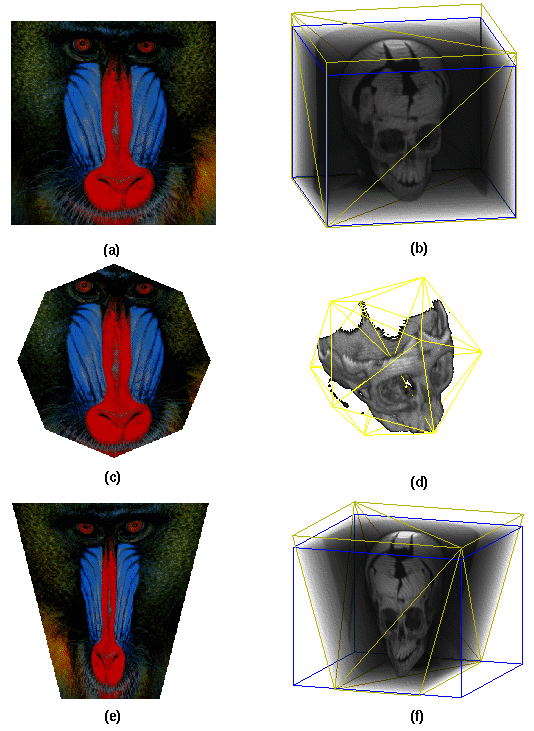• A two-dimensional array of pixels mapped onto a matching size rectangle, (a), is similar to a three-dimensional array of voxels mapped onto a matching size cube, (b).

• An octagonal “cookie-cutter” used to focus on a portion of the texture, (c), is similar to an icosahedral geometry used to focus on a portion of a volume, (d).

• A rectangular texture mapped onto an arbitrary quadrangle and the resulting distortion, (e), is similar to a regular volume mapped onto an irregular geometry and the resulting distortion, (f).

### Advantage of Separating Geometry and Appearance

Because geometry and appearance are defined independently of one another in OpenGL Volumizer, you never need to write special code that changes the rendering engine for special features, such as:

• Arbitrarily-shaped volumes of interest (VOIs)

Applications can choose to render only a portion of the volume, for example, a sub-cube or a spherical region containing interesting features. To do this you pass a different set of primitives (tetrahedra) as a parameter to the renderer. No changes to the renderer itself are needed.

• Volume deformation

You can change a shape by changing its volume but without changing its voxels. This three-dimensional deformation is analogous to stretching a texture-mapped polygon. Figure 2-5 shows how a cube is transformed into a truncated pyramid.

Figure 2-5. Volume Deformation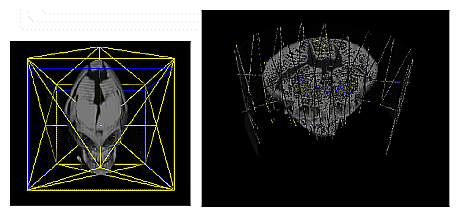The left panel in Figure 2-5 shows a simple free form deformation application in which the vertices defining the volume's geometry are moved around affecting the shape of the model. The right panel illustrates a contrained deformation: the geometry is distorted radially. This feature can prove useful in applications that deal with ultrasonic and radar data, which need to be “dewarped.”

• Hierarchical modeling

Applications can create tessellations that define sub-parts within shapes. For example, rather than use a canonical five-tetrahedron tessellation of a volume, for example a skull, a separate set of tetrahedra can be specified to model the jaw, or a bone flap. These subsections can be manipulated as separate objects (with different material properties and transformations) to simulate maxillofacial or brain surgery.

Figure 2-6. Jaw Modeled as a Separate Part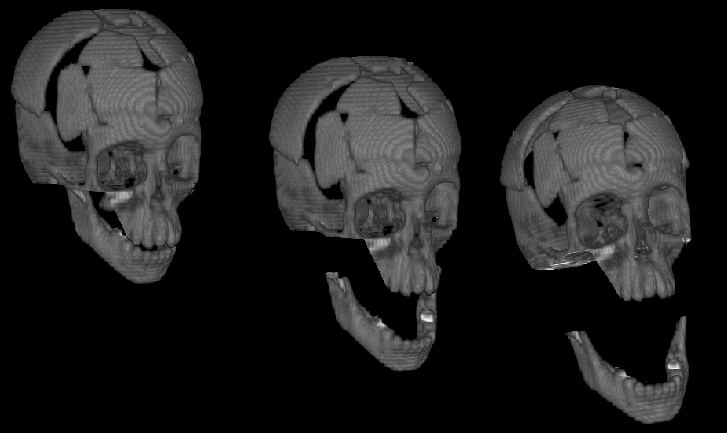• Per-part appearance.

Applications can assign different properties (e.g., colors) to highlight or otherwise distinguish individual subparts of the model. This can be useful in labeling geological material in a seismic data interpretation application or for diagnostic data set segmentation.

Figure 2-7. Per-part Properties.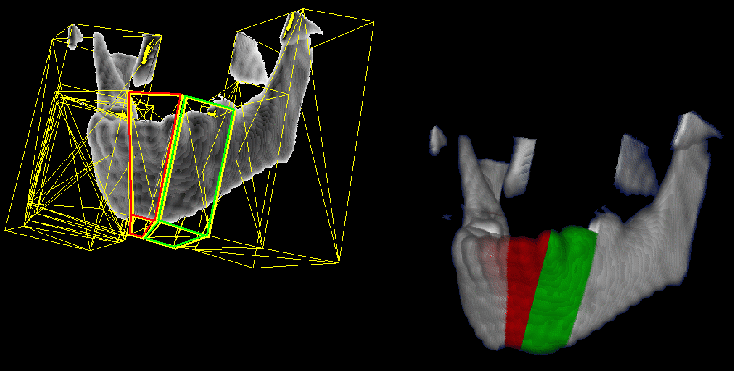• Space leaping techniques

You can use a very fine tessellation to produce a large number of small cubes to skip areas of void in the scene (analogous to polygon assisted ray casting (PARC) and other space-leaping techniques) in a simple pre-processing step, as shown in Figure 2-8.

Figure 2-8. Finely Tessellated Shape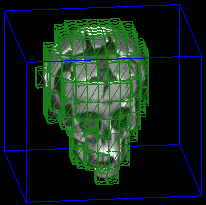This technique, called space leaping, can sometimes produce a dramatic performance increase for sparse data sets by reducing pixel fill calculations.

### Tetrahedron as a Primitive

The triangle is the simplest and most flexible primitive you can use to represent polygonal geometry; any surface can be decomposed into a collection of triangles to approximate the surface of a geometry. A circle, for example, can be approximated by thirty triangles all sharing a common vertex in the center of the circle.

Similarly, a tetrahedron is the simplest and most efficient primitive you can use to represent volumetric geometry. Any shape can be tessellated into tetrahedra; for example, any cube can be decomposed into as few as five tetrahedra, as shown in Figure 2-9.

Figure 2-9. Tetrahedral Decomposition of Cubic Geometry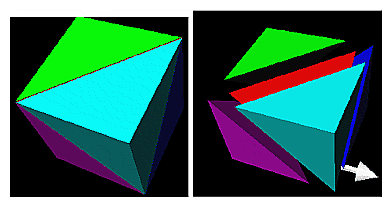Or, for example, an icosahedron (a rough approximation of a sphere) can be tessellated into 20 tetrahedra by connecting the center of the icosahedron with triangles on the surface, as shown in Figure 2-10.

Figure 2-10. Tessellation of Spherical Geometry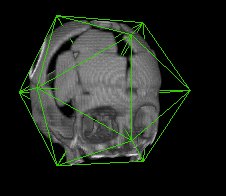#### Higher-Level Primitives

At times it is more convenient to specify higher-level geometric shapes, such as boxes or solid spheres, as primitives. You might use a higher-level primitive, for example, when using complex-shaped volumes of interest, for example, a logo of the company filled with smoke. Some of the polyhedral primitives are shown in Figure 2-11.

Figure 2-11. Volumetric Primitives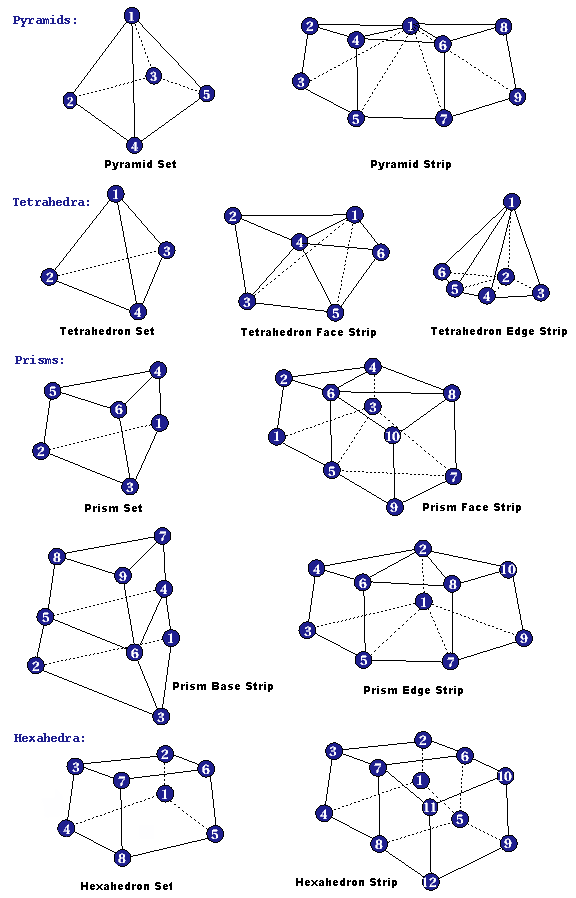## Volume Rendering Techniques

There are many traditional ways to render a volume, including ray casting, splatting, and shear warp. OpenGL Volumizer uses a technique, similar to ray casting, called volume slicing, to leverage the texture-mapping hardware many workstations now have.

### Ray Casting vs. Volume Slicing

Ray casting can be performed in ray-order or sampling-surface order using planes or spherical surfaces parallel to the line of sight, as shown in Figure 2-12.

Figure 2-12. Processing Order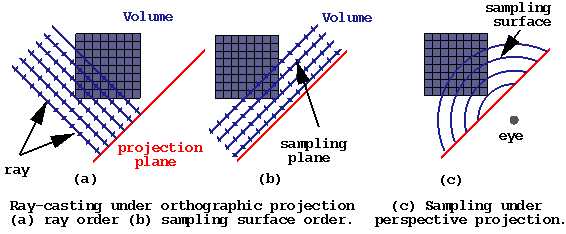Volume slicing and ray casting are equivalent in the following ways:

• Ray casting under orthographic projection ((a) and (b) in Figure 2-12) is equivalent to taking a series of slices along planes parallel to the viewport and compositing them.

• Ray casting under perspective projection ((c) in Figure 2-12) is equivalent to sampling along a series of concentric spherical shells centered at the eye.

The end result is volume rendering according to texture mapping, as shown in Figure 2-13.

Figure 2-13. Volume Rendering as Texture Mapping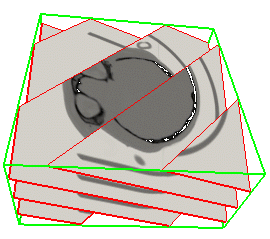#### Ray Casting Processing Order

In ray casting, each point on a ray projected from the eye position through the volume is processed sequentially. In this technique:

1. The colors, opacity and shading of a volume are sampled, filtered, and accumulated at a point on a ray a specific distance from the eye position.

2. The distance is incremented along the ray, and the same colors, opacity and shading computations are repeated by the CPU, as shown in Figure 2-14.

Figure 2-14. Ray Casting Processing order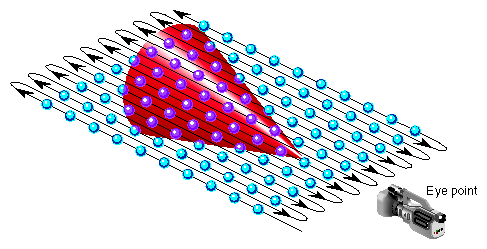3. When the traversal finally extends beyond the viewing frustum, the point of computation moves to the next ray near the eye point.

Conventionally, the main processing loop operates in ray order.

#### Volumizer Processing Order

In Volumizer, all points on a plane orthogonal to the line of sight are computed sequentially in the texture mapping hardware. In this technique:

1. The volume is sampled in surfaces orthogonal to the viewing direction, as shown in Figure 2-15.

Figure 2-15. Volume Slicing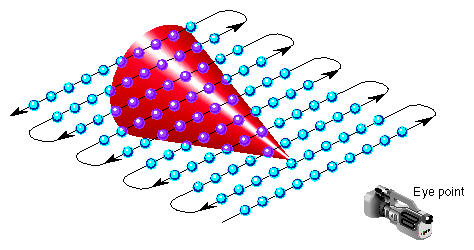2. After the points along one plane on all the rays intersecting the volume are processed, the distance is incremented and the processing occurs again for all points on all the rays in the new plane.

3. Processing the points continues until the plane of points moves beyond the viewing frustum, at which point the processing terminates.

The results of ray casting and volume slicing are identical. Orthographic projection is equivalent to volume slicing using sampling planes, and perspective ray casting is similar to using sampling along generalized (non-planar) surfaces in Volumizer.

There are, however, some important differences between the two techniques in processing the volumes:

• Volume slicing is faster than ray casting because computations are performed by the dedicated texture mapping hardware, whereas ray casting computations are performed by the CPU.

• Volume slicing reduces the volume to a series of texture-mapped, semi-transparent polygons. These polygons are in no way special and can be merged with any other polygonal data base handed to any graphics API (for example, OpenGL or Optimizer) for drawing.

## Using Minimal Tessellations

In many common examples, volumes are hexahedral. The minimum tessellation of a hexahedron, as shown in Figure 2-9, is five tetrahedra. There are, however, advantages and disadvantages to using minimal tessellations.

When voxel coordinates and vertex coordinates coincide, you can use a single, large tetrahedron that fully encloses a voxel array to increase performance. For example, given a voxel array of SIZE3, you would use a tetrahedron with vertices at (0, 0, 0), (3 × SIZE, 0, 0), (0, 3*SIZE, 0), and (0, 0, 3 × SIZE). Such a single-tetrahedron tessellation can reduce polygonization calculations by a factor of five.

While compact, a minimal tessellation is not uniform and tends to introduce interpolation artifacts. For example, Figure 2-16 shows how colors are not uniformly interpolated across a face.

Figure 2-16. Non Uniform Interpolation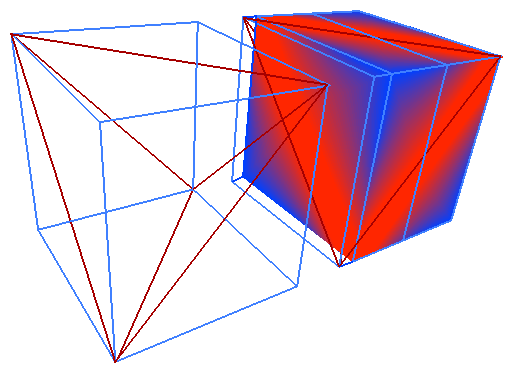The vertices of the cube alternate between red (bright) and blue (dark). One would expect the faces of the cube to be smoothly interpolated between the respective vertices. However, the interpolation only occurs within a tetrahedron. Therefore, the resulting faces will have either a red (bright) or a blue (dark) diagonal band running along the edge of the tetrahedron that divides them. This artifact is analogous to creating T-junctions in polygonal tessellations.

Connecting multiple boxes through face adjacency leads to inconsistent (and highly noticeable) interpolation bands. For example, the wire frame box in Figure 2-16 has the same tessellation and vertex coloring as the solid one.

Yet, due to the asymmetry inherent in the tessellation, the adjacent faces have opposite diagonals as bases for their tessellations. Therefore, one of the two adjacent faces (solid box) is rendered with red (bright) running along one diagonal, while the other (wire frame box) has a similar band running along the opposite diagonal.

Worse yet, moving one of the vertices shared between two adjacent boxes results in “cracking.”

These artifacts make the use and manipulation of complex volumetric primitives cumbersome.

All of the artifacts discussed can be minimized or avoided by splitting the cube into a larger number of more uniformly distributed tetrahedra. For example, it is possible to split a cube into 6 pyramids with the apex in the center of the cube using the faces of the cube as their bases. These pyramids can be further subdivided into four tetrahedra each for a total of 24 tetrahedron.

## Virtualizing Voxel Memory

Some image data bases contain more voxel data than can be stored in a machine's texture mapping hardware. To take advantage of hardware acceleration, voxel data is broken up into subsections, called bricks.

### Bricks

A brick is a subset of voxel data that can fit into a machine's texture mapping hardware. Bricks are regular hexahedra (boxes) with non-zero width, height, and depth. Displaying a volume requires paging the appropriate brick data into texture memory. Anticipating which bricks to page can speed up your application's performance.

Applications have control over individual bricks; for example, it is an application's responsibility to provide voxel data for each brick.

Bricks are three-dimensional objects but can also be used to represent two-dimensional textures by setting one of the dimensions to 1. This way stacks of 2D images can easily be handled.

### Brick Sets

One or more adjacent bricks constitute a brick set. Volumetric appearance is defined as a collection of one or more brick sets. Figure 2-17 shows a brick set containing eight bricks, shown as eight cubes within the large cube.

Figure 2-17. Brick Set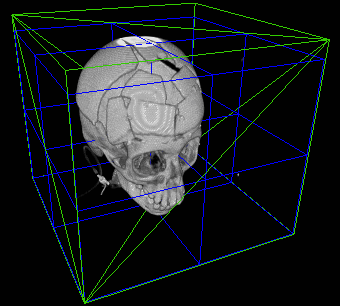#### Brick Set Collections

Typically, a shape can be described by a single brick set. In certain situations, however, more than one brick set is required. For example, on machines that do not support three-dimensional texture mapping, three separate copies of the data set may have to be maintained, one for each major axis, as shown in Figure 2-18, to minimize sampling artifacts.

Figure 2-18. Data Replication for Two-dimensional Texturing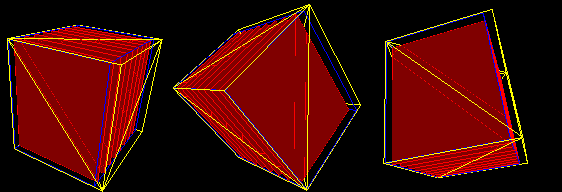### Brick Overlap

Bricks typically have to overlap to prevent seams from appearing at the brick boundaries.

Figure 2-19. Brick Overlap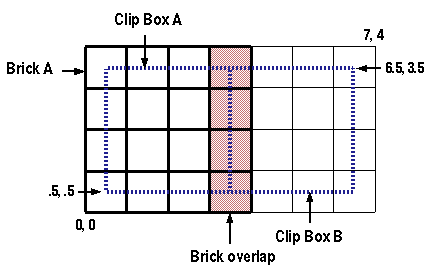Figure 2-19 shows that bricks overlap but clip boxes, described in “Clip Boxes”, do not; they are adjacent. the difference in size between the brick and the clip box determines the brick overlap which helps prevent artifacts, such as seams, in the image.

The amount of overlap depends on the filtering scheme used. For example, if an application requested:

• Tri-linear interpolation, the bricks have to overlap by a layer of voxels one voxel thick in each direction

• Cubic interpolation, three layers are needed in each direction

Another factor that determines the width of brick overlap is gradient computation. When the gradient is computer, the x, y, and z dimensions for each voxel are graded over x + 1 to x - 1, y + 1 to y - 1, and z + 1 to z - 1. If any of these values fall outside the brick, the overlap might be greater.

Overlaps create storage overhead that is typically negligible, but may be substantial in certain situations. For example, requesting that a volume divided into bricks that are one voxel thick and insisting on 1 voxel overlap in all directions triples the storage overhead.

#### Clip Boxes

Clip boxes are used to clip geometry that spans several bricks in a way that guarantees that individual pieces join seamlessly. A clip box is centered where a brick is, but is smaller by the amount of overlap. Clip boxes of adjacent bricks are strictly adjacent; that is, there is no overlap or void between them.

Figure 2-20 illustrates the spatial relationships for two 4-by-4 bricks overlapping by one layer of voxels. The clip boxes are represented by the dotted lines.

Figure 2-20. Bricks Overlapping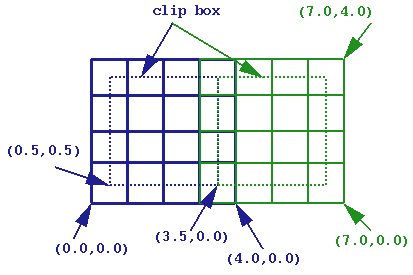It is convenient to associate a clip box with a brick. A brick is characterized by its origin and its size. In Figure 2-20, the brick on the left has its origin at (0,0). The brick on the right has its origin at (3,0). The left and right clip box origins are (0.5, 0.5) and (3.5, 0.5), respectively.

#### Brick Size Restriction

Brick sizes are required to be a power of 2. This restriction allows implementations to take advantage of the underlying graphics API, OpenGL. This requirement does not mean, however, that the volume itself has any size restrictions.

If the volume dimensions do not divide evenly into brick dimensions, you can do one of the following:

• Ignore the voxels that fall outside of the brick set that evenly divides the volume.

For example, a 256 × 256 × 190 volume bricked into 1283 bricks with one voxel overlap in each direction creates 8 bricks discarding a single layer of voxels in the X and Y directions, and 62 layers in the Z direction (Figure 2-12).

• Add an additional layer of bricks in each direction that are partially empty.

In the previous example, that would mean creating 27 × 1283 bricks.

• Try to make the brick as small as possible: determine the smallest power of 2 that fits the partial brick.

In the previous example, that would result in creating 27 bricks, but the X and Y dimensions of the partial bricks would be 2, and the Z dimension would be 64. This means that bricks may be of sizes other than the one requested.

## Polygonizing Shapes

To render a shape, Volumizer slices a volume along a set of parallel surfaces; this slicing process is called polygonization. The result is a set of polygons, called faces. Textures are associated with each of these polygons, as shown in Figure 2-21.

Figure 2-21. Polygonal Slices of a Tetrahedron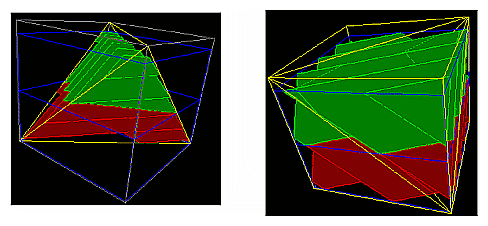The figure on the left in Figure 2-21 shows the polygonization of a tetrahedron. The figure on the right shows the polygonization of five tetrahedra that define a cube.

The orientation of the surfaces is configurable; the simplest case is when the surfaces are orthogonal to the line of sight of the viewer. The surfaces, however, can be aligned arbitrarily.

The slices in these figures are planar; they can, however, be of any shape. In particular, you might choose to shape them spherically to render equidistant points from the viewpoint.

### Faces Clipped to Brick Boundaries

Polygonization clips polygons to brick and volume boundaries to facilitate texture paging. Figure 2-22 shows a single tetrahedron spanning two bricks, which are stacked vertically.

Figure 2-22. Face Sets Spanning Two Bricks Stacked Vertically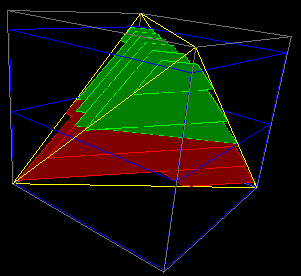Figure 2-22 shows a separate set of polygons for each brick. polygonization() depth sorts these polygons and hands them to the application for drawing.